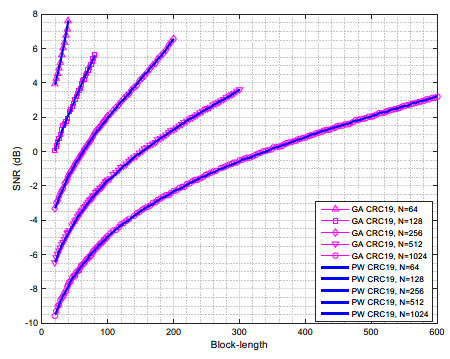# 预备知识

## 通用偏序（UPO）

Addition：若一个合成信道索引用二进制表示为$$\left( a,b,1,c \right)$$，那么它的可靠性一定大于合成信道$$\left( a,b,0,c \right)$$$$''1\succ 0''$$模式对于1比特或多比特同样适用。例如：

2（0, 1 ,0） $$3（0, 1, 1） 9（1, 0, 0, 1）$$ 15（1, 1, 1, 1）

Left-swap：若合成信道索引为$$\left( a,0,b,1,c \right)$$，那么它的可靠性一定小于合成信道$$\left( a,1,b,0,c \right)$$$$''0...1\prec 1...0''$$模式可以出现多次，并且0,1不需要彼此相邻。例如：

2（0, 1 ,0） $$4（1, 0, 0） 12（0, 1, 1, 0, 0）$$ 24（1, 1, 0, 0, 0）

3（0, 1, 1）and 4（1, 0, 0）

7（0, 1, 1, 1）and 12（1, 1, 0, 0）

# UPO的性质

Proposition 1. （嵌套和对称）极化码通用偏序有两个重要性质：

# β-expansion：PW算法原理

\begin{align} { {f}^{PW}}:x\mapsto \sum\limits_{i=1}^{n}{ { {b}_{i}}{ {\beta }^{i}}} \end{align}

${ {w}_{3}}=0\cdot { {2}^{ {3}/{4}\;}}+0\cdot { {2}^{ {2}/{4}\;}}+1\cdot { {2}^{ {1}/{4}\;}}+1\cdot { {2}^{ {0}/{4}\;}}=2.189\cdot \cdot \cdot$

\begin{align} \nonumber & w=\left\{ \begin{matrix} 0.000 & 1.000 & 1.189 & 2.189 & 1.414 & 2.414 & 2.603 & 3.603 \\ \end{matrix} \right. \\ \nonumber & \ \ \ \ \ \ \ \left. \begin{matrix} 1.682 & 2.682 & 2.871 & 3.871 & 3.096 & 4.096 & 4.285 & 5.285 \\ \end{matrix} \right\} \\ \end{align}

$${ {w}_{x}}$$值越大表示合成信道索引$$x$$的可靠性越高。将$$w$$按可靠性增序排列，就得到了极化码序列

$order=\left\{ \begin{matrix} 0 & 1 & 2 & 4 & 8 & 3 & 5 & 6 & 9 & 10 & 12 & 7 & 11 & 13 & 14 & 15 \\ \end{matrix} \right\}$

PW算法的优点是，对合成信道可靠性，提供了一种简洁、低复杂度的全排列算法，并且当码长$${ {2}^{n}}$$$$n=1$$到$n+$增加时，能够始终保持一种嵌套性质。

Proposition 2. （嵌套结构）PW算法维持了极化码的嵌套码构造的特性。

Proposition 3.$$\beta >1$$，任何由PW算法得到的序列都遵循极化码的通用偏序（UPO）关系。

Theorem 1. 对于长度为$${ {2}^{n}}\left( n\ge 3 \right)$$的极化码，存在一个升序集合$${ {A}_{n}}=\left\{ { {a}_{0}},{ {a}_{1}},{ {a}_{2}},...,{ {a}_{ { {i}_{\max }}}},+\infty \right\}$$，其中$${ {a}_{0}}=1$$$${ {a}_{1}},{ {a}_{2}},...,{ {a}_{ { {i}_{\max }}}}$$都是代数。任意$$$落入区间$$( { {a}{i}},{ {a}{i+1}} ),i$$内，β-expansion都是唯一的，并且$$$$落入不同区间可导致不同的顺序序列。此外，集合$${ {A}{n}}$$是嵌套的，即$${ {A}{n}}$。

Marshall：通过上述Proposition和Theorem，初步地把β-expansion和偏序建立起联系，β-expansion获得了偏序的理论支撑。两者的共性在于，它们都具有嵌套结构。然而$$的值究竟取何值呢？这还需要在具体的用例中去分析和确定。 # AWGN信道下的快速构造 现在UPO已经成为极化码序列构造的基础，而极化码序列的构造决定了合成信道的可靠性顺序。但UPO无法做到对整个序列进行全排列，正如在UPO的性质一节所看到的，从$$\mathbf{UP}{ {\mathbf{O}}_{n-1}}$$$$\mathbf{UP}{ {\mathbf{O}}_{n}}$$的构造中，总会有一些新的序是UPO无法判断的。而高斯近似虽然能够对整个序列进行全排序，但由于其高复杂度而不适用于实际应用。为了克服两者的缺点，本节呈现了一种基于UPO和β-expansion的快速构造方法。 Marshall：根据高斯近似的结果，一步一步缩小$$的取值范围，最终的序列不仅满足UPO性质，而且与高斯近似的结果保持一致。换句话说，没有条件，就去创造条件。

\begin{align} \nonumber & 28\prec 15,\ 24\prec 11\Leftrightarrow \beta <1.221 \\ \nonumber & \ \ \ \ \ \ \ \ \ \ \ \ 14\prec 19\Leftrightarrow \beta <1.325 \\ \nonumber & \ \ \ \ \ \ \ \ \ \ \ \ 24\prec 13\Leftrightarrow \beta <1.272 \\ \end{align}

$7\prec 24\Leftrightarrow \beta >1.179$

# 渐进性分析与数值仿真结果

β-expansion可以转化为贝努力卷积（Bernoulli Convolution）概念。贝努力卷积定义了概率测度

${ {v}_{\lambda }}=\sum\limits_{i=1}^{+\infty }{ { {b}_{i}}}{ {\beta }^{i}}$

$\Pr \left\{ { {b}_{i}}=1 \right\}=\Pr \left\{ { {b}_{i}}=0 \right\}=0.5,\forall i$# 参考文献

 C. Schurch, “A partial Order for the Synthesized Channels of a Polar Code, ISIT 2016.  3GPP, R1-167209, Polar code design and rate matching, Huawei, HiSilicon.  β-expansion A Theoretical Framework for Fast and Recursive Construction of Polar Codes, Huawei.